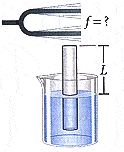# Tuning fork and harmonics

• lettertwelve
In summary, the frequency of a tuning fork can be found by adjusting the length of an air column in a submerged tube until sound intensity is at a peak. The smallest value for this length is 8.00 cm with a speed of sound in air of 330 m/s. In part (a), the question asks for the frequency of the tuning fork, which can be calculated using the given information. In part (b), the question asks for the value of L for the next two harmonics, which can be found by using the equation V/4L. However, it is important to use the correct units in the calculation and to carefully check for any errors.

## Homework Statement

The frequency of a tuning fork can be found by the method shown in Figure 13-24. A long tube open at both ends is submerged in a beaker of water, and the vibrating tuning fork is placed near the top of the tube. The length of the air column, L, is adjusted by moving the tube vertically. The sound waves generated by the fork are reinforced when the length of the air column corresponds to one of the resonant frequencies of the tube. The smallest value for L for which a peak occurs in sound intensity is 8.00 cm. (Use 330 m/s as the speed of sound in air.)(a) What is the frequency of the tuning fork?
______Hz
(b) What is the value of L for the next two harmonics?
______m
______m

V/4L ?

## The Attempt at a Solution

i tried the V/4L for part b, but nothing works.
i don't know where to even start

Hi lettertwelve,

What numbers did you use in your attempts for part b? Did you find the frequencies of the next two harmonics?

•arimariewil22
Not only what numbers did you use, but did you include units in the calculation?

What did you get when you calculated V/4L, and how do you know (or why do you think) it is wrong?